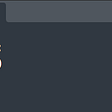# Passing a callback function from Python to C

To communicate between python and C, we have a library `ctypes`

Step — 1 : create a c program, in which one function receives another function pointer as parameter.

In the below example, the function `divide` receives another function pointer as first parameter, which receives 2 parameters, quotient and remainder.

save the program as `division.c`

`#include <stdio.h>void divide(void (*ptr)(int *, int *), int a, int b){  int s = a / b;  int r = a % b;  (*ptr) (&s, &r);}void print_sum(int * s, int * r){ printf("Quotient is %d, remainder is %d\n", *s, *r);}int main(){  void (*ptr)() = &print_sum;  divide(ptr, 7, 4);}`

To run the above program

\$gcc division.c

\$./a.out

Now create a shared library

\$gcc -fPIC -shared -o libdiv.so division.c

Step — 2 : Create a python program

`from ctypes import CFUNCTYPEfrom ctypes import c_void_pfrom ctypes import POINTERfrom ctypes import c_intfrom ctypes import cdlllib = cdll.LoadLibrary('./libdiv.so')CMPFUNC = CFUNCTYPE(c_void_p, POINTER(c_int), POINTER(c_int))def py_cmp_func(s, r):  print (f'Quotient is {s} , remainder is {r}')cmp_func = CMPFUNC(py_cmp_func)lib.divide(cmp_func, 3, 5)`

save the program as test.py in the same directory

Now run it

\$python3 test.py

You will get the following output

Quotient is 0 , remainder is 3

--

--

--

## More from Prince Francis

Providing simple solutions for complex problems.

Love podcasts or audiobooks? Learn on the go with our new app.

## Boost up infrastructure while saving money - Part I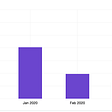## MLOps vs DevOps. What’s the difference?## The perils of functional CSS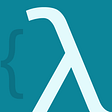## Simple Guide to Laravel Authorization with Gates## 30 Days Coding Challenge — Day 4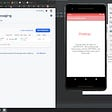## CheerpX for Flash now generally available## Quick Fun Speed Lab## InsureFi — HackMoney 2022 Buidl## Prince Francis

Providing simple solutions for complex problems.

## 5 Interesting Numpy Functions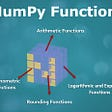## Pattern 2: Two Pointers## The Python Series #4 — While Loop and General Terminologies